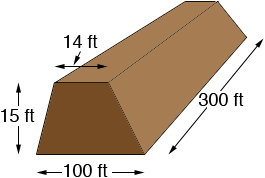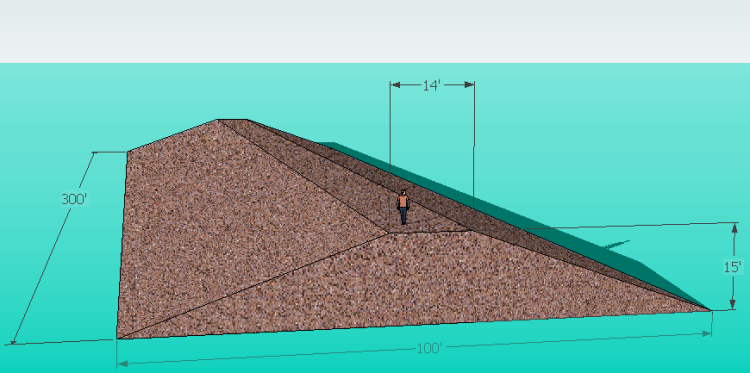SEARCH HOMEMath Central Quandaries & QueriesQuestion from JD How many cubic yards of soil are required to build a small Pond Dam: 100ft wide bottom x 300ft long x 15ft High with a 14ft wide Flat topWe have two responses for you

Hi,

My diagram is not to scale.The volume of the dam is the area of the trapezoidal end times the length. The area of a trapezoid is the average of the lengths of the parallel sides times the distance between the parallel sides. Since you want the volume in cubic yards I would change all the dimensions to yards before making the calculations.

Area of the trapezoid: [(100/3) +( 14/3)]/2 × 15/3 = 38/2 × 5 = 95 square yards.

Volume of the dam: 95 × 300/3 = 9500 cubic yards.

Harley

Hi there.

The shape you are describing sounds like the shape in this diagram:Its volume is the area of the trapezoid ABCD times the length. The area of any trapezoid is the average of the top and bottom widths times the height. So the area of yours is (100 + 14) / 2 x 15 = 855 square feet. Thus the volume of your dam is 855 x 300 = 256500 cubic feet.

To convert feet to yards you divide by 3, so to convert cubic feet to cubic yards, you divide by 33 = 27.

256500 / 27 = 9500 cubic yards of soil. This assumes no compaction, of course.

Cheers,
Stephen La Rocque.Math Central is supported by the University of Regina and The Pacific Institute for the Mathematical Sciences.